# GFR Calculator## Glomerular Filteration Rate (GFR)

Glomerular filtration rate (GFR) is the best overall index of kidney function. Normal GFR varies according to age, sex, and body size, and declines with age. Glomerular filtration rate (GFR) is the volume of fluid filtered from the renal (kidney) glomerular capillaries into the Bowman's capsule per unit time.

Glomerular filtration rate (GFR) can be calculated by measuring any chemical that has a steady level in the blood, and is freely filtered but neither reabsorbed nor secreted by the kidneys. The rate therefore measured is the quantity of the substance in the urine that originated from a calculable volume of blood. The GFR is typically recorded in units of volume per time, e.g., milliliters per minute ml/min. Compare to filtration fraction.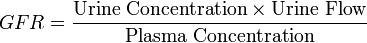There are several different techniques used to calculate or estimate the glomerular filtration rate (GFR or eGFR). The Most recent techniques are CKD EPI and MDRD GFR Formula. The details of these GFR Calculation formulas can be see after the calculator. The following GFR Calculator is based on these new GFR Calculation techniques.

## CKD EPI & MDRD GFR Calculator

NOTE: CKD EPI GFR is only valid with creatinine methods are traceable to IDMS
years
Race
Gender
TRACEABLE TO IDMS*

## Auto GFR Calulation

CKD EPI Value: epi

MDRD GFR: mdrd

(Age, Race, Gender, Plasma creatinine)

* Isotope dilution mass spectrometry (IDMS) reference measurement procedure.

*All ethnic groups other than African American

### Estimated GFR (eGFR) using Modification of Diet in Renal Disease (MDRD) formula

The most recently advocated formula for calculating the GFR is the one that was developed by the Modification of Diet in Renal Disease Study Group. Most laboratories in Australia, and The United Kingdom now calculate and report the MDRD estimated GFR along with creatinine measurements and this forms the basis of Chronic kidney disease#Staging. The adoption of the automatic reporting of MDRD-eGFR has been widely criticised.

The most commonly used formula is the "4-variable MDRD," which estimates GFR using four variables: serum creatinine, age, race, and gender. The original MDRD used six variables with the additional variables being the blood urea nitrogen and albumin levels. The equations have been validated in patients with chronic kidney disease; however both versions underestimate the GFR in healthy patients with GFRs over 60 mL/min. The equations have not been validated in acute renal failure.

For creatinine in mg/dL: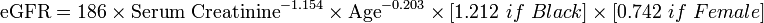For creatinine in µmol/L: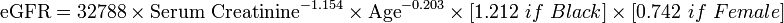Creatinine levels in µmol/L can be converted to mg/dL by dividing them by 88.4. The 32788 number above is equal to 186×88.41.154.

A more elaborate version of the MDRD equation also includes serum albumin and blood urea nitrogen (BUN) levels: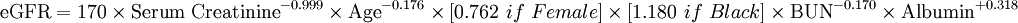Where the creatinine and blood urea nitrogen concentrations are both in mg/dL. The albumin concentration is in g/dL.

These MDRD equations are to be used only if the laboratory has NOT calibrated its serum creatinine measurements to isotope dilution mass spectrometry (IDMS). When IDMS-calibrated serum creatinine is used (which is about 6% lower), the above equations should be multiplied by 175/186 or by 0.94086.

Since these formulae do not adjust for body mass, they (relative to the Cockcroft-Gault formula) underestimate eGFR for heavy people and overestimate it for underweight people. (see Cockcroft-Gault formula above).

### Estimated GFR (eGFR) using the CKD-EPI formula

The CKD-EPI (Chronic Kidney Disease Epidemiology Collaboration) formula was published in May 2009. It was developed in an effort to create a formula more accurate than the MDRD formula, especially when actual GFR is greater than 60 mL/min per 1.73 m2.

Researchers pooled data from multiple studies to develop and validate this new equation. They used 10 studies that included 8254 participants, randomly using 2/3 of the data sets for development and the other 1/3 for internal validation. Sixteen additional studies, which included 3896 participants, were used for external validation.

The CKD-EPI equation performed better than the MDRD (Modification of Diet in Renal Disease Study) equation, especially at higher GFR, with less bias and greater accuracy. When looking at NHANES (National Health and Nutrition Examination Survey) data, the median estimated GFR was 94.5 mL/min per 1.73 m2 vs. 85.0 mL/min per 1.73 m2, and the prevalence of chronic kidney disease was 11.5% versus 13.1%.

The CKD-EPI equation, expressed as a single equation, is: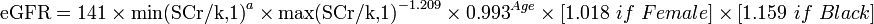where SCr is serum creatinine (mg/dL), k is 0.7 for females and 0.9 for males, a is -0.329 for females and -0.411 for males, min indicates the minimum of SCr/k or 1, and max indicates the maximum of SCr/k or 1.

A clearer version may be as follows: For creatinine (IDMS calibrated) in mg/dL:
African American Female
If serum creatinine (Scr) <=0.7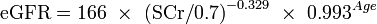If serum creatinine (Scr) > 0.7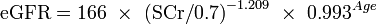African American Male
If serum creatinine (Scr) <=0.9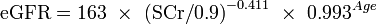If serum creatinine (Scr) > 0.9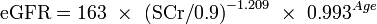White or other race Female
If serum creatinine (Scr) <=0.7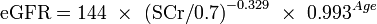If serum creatinine (Scr) > 0.7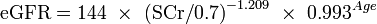White or other race Male
If serum creatinine (Scr) <=0.9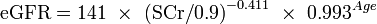If serum creatinine (Scr) > 0.9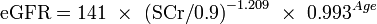This formula was developed by Levey et al.

The formula CKD-EPI may provide improved cardiovascular risk prediction over the MDRD Study formula in a middle-age population.

You can either use these GFR calculation formula's or use our GFR Calculator above to automatically calculate your Glomerular Fileration Rate ior GFR.